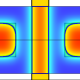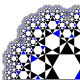# The truth about polynomial factorization

In yesterday’s blog, called The Fundamental Dream of Mathematics, I started to explain why modern mathematics is occupying a Polyanna land of wishful dreaming, with its over-reliance on the FTA — the cherished, but incorrect, idea that any non-constant polynomial p(x) has a complex zero, that is there is a complex number z satisfying p(z)=0. As a consequence, we all happily believe that every degree n polynomial has a factorization, over the complex numbers.

What a sad piece of delusional nonsense this is: a twelve year old ought to be able to see that we are trying to pull the wool over our collective eyes here. All what is required is to open up our computers and see what really happens!

Let’s start by looking at a polynomial which actually is a product of linear factors. This is easy to cook up, just by expanding out a product of chosen linear factors:

p(x)=(x-3)(x+1)(x+5)(x+11)= x⁴+14x³+20x²-158x-165.

No-one can deny that this polynomial does have exactly four zeroes, and they are x=3,-1,-5, and -11. Corresponding to each of these zeroes, there is indeed, just as Descartes taught us, a linear factor. If I don’t tell my computer where the polynomial is coming from, and just ask it to factor p(x)=x⁴+14x³+20x²-158x-165, then it will immediately inform me that

x⁴+14x³+20x²-158x-165= (x+11)(x-3)(x+5)(x+1).

Now let’s modify things just a tad. Let’s change that last coefficient of -165 to -166. So now if I ask my computer to factor q(x)=x⁴+14x³+20x²-158x-166, then it will very quickly tell me that

x⁴+14x³+20x²-158x-166= -158x+20x²+14x³+x⁴-166.

This is the kind of thing that it does when it cannot factor something. Did I tell you that my computer is very, very good at factoring polynomial expressions? I have supreme confidence in its abilities here.

But wait a minute you say, clearly your computer is deluded Norman! We know this polynomial factors, because all polynomials do. That is what the Fundamental Theorem of Algebra asserts, and it must be right, because everyone says so. Why don’t you find the zeroes first?

Okay, let’s see what happens if we do that: if I press solve numeric after the equation

x⁴+14x³+20x²-158x-166=0

the computer tells me that: Solution is: {[x=-1. 006254],[x=-4. 994786],[x=-11. 00119],[x=3. 002230]}

But these are not true zeroes, as we saw in yesterday’s blog, they are only approximate zeroes. True zeroes for this polynomial in fact do not exist.

True zeroes for this polynomial in fact do not exist.

True zeroes for this polynomial in fact do not exist.

Probably I will have to repeat this kind of mantra another few hundred times before it registers in the collective consciousness of my fellow pure mathematicians!

Let us check if we do get factorization: we ask the computer to expand

(x+1.006254)(x+4.994786)(x+11.00119)(x-3.002230)

and it does so to get

x⁴+14.0x³+20. 00000x²- 158.0x-166.0.

Hurray! We have factored our polynomial successfully! [NOT]

Here is the snag: the coefficients are given as decimal numbers, not integers. That means there is the possibility of round-off. Let me up the default number of digits shown in calculations from 7 to 20 and redo that expansion. This time, I get

x⁴+14.0x³+19. 999999656 344x²- 158. 000005080 13515776x-166. 000016519 11547081.

Sadly we see that the factorization was a mirage. Ladies and Gentlemen: a polynomial that does not factor into linear factors: q(x)=x⁴+14x³+20x²-158x-166.

Here is the true story of rational polynomial factorization: polynomials which factor into linear factors are easy to generate, but if you write down a random polynomial with rational coefficients of higher degree, the chances of it being of this kind are minimal. There is a hierarchy of factorizability of polynomials of degree n, whose levels correspond to partitions of n. For example if n=4, then there are five partitions of 4, namely 4, 3+1, 2+2, 2+1 and 1+1+1+1. Each of these corresponds to a type of factorizability for a degree four polynomial.

Here are example polynomials that fit into each of these kinds:

x⁴+14x³+20x²-158x-166=(x⁴+14x³+20x²-158x-166)

x⁴+14x³+21x²-147x-165= (x³+3x²-12x-15)(x+11)

x⁴+14x³+18x²-172x-216= (x²-2x-4)(x²+16x+54)

x⁴+14x³+19x²-156x-162= (16x+x²+54)(x-3)(x+1)

x⁴+14x³+20x²-158x-165= (x+11)(x-3)(x+5)(x+1).

So the world of polynomial factorizability is much richer than we pretend. But that also means that the theory of diagonalization of matrices is much richer than we pretend. The theory of eigenvalues and eigenvectors of matrices is much richer than we pretend. And many other things besides.

In distant times, astronomers believed that all celestial bodies moved around on a fixed celestial sphere centered on the earth. What a naive, convenient picture this was. In a thousand years from now, that is the way people will be thinking about our view of algebra: a simple-minded story, useful in its way, that ultimately just didn’t correspond to the way things really are.

## 9 thoughts on “The truth about polynomial factorization”

1.Jonathan Crabtree

Another interesting post Norman.

The Pythagoreans discovered a similar crisis around 2600 years ago when they couldn’t describe the diagonal of a unit square as a ratio of two numbers. They found some numbers can’t be expressed as simple integral ratios and today, we describe those numbers un-ratio-able or irrational.

The fact a polynomial curve crosses the x axis at a point that can’t be expressed as a ratio of two whole numbers doesn’t mean the equation does not have any roots. It may just mean most roots of randomly generated polynomials with integral coefficients are irrational, as might be expected.

Why can’t we simply let the factors of an equation be non-commensurable?

Euclid and Descartes may have missed it, yet multiplication on the reals (including the irrationals) can be solved with a compass and straight edge construction, as revealed at: http://education.lms.ac.uk/2015/04/jonathan-crabtree-multiplication-on-the-reals-with-a-circle/

Am I right in assuming any infinite series that approximates an irrational is also bad mathematics?

Jonathan

2.gentzen

I sometimes wonder whether you are aware of the historical context, which led Cantor to found the DMV (Deutsch Mathematiker Vereinigung), and maintain the idea that logical consistency is the only touchstone for a mathematical theory. But I wouldn’t really blame Cantor or Hilbert for the modern fairytale mathematics. My guess is rather that people like Alfred Tarski intentionally created it, and that they knew exactly what they were doing. Modern axiomatic mathematics certainly oversimplifies things too much, but it provides a common language and a common ground enabling effective communication of mathematical ideas. Tarski’s theorem about choice is a good example of how communication can break down without such a common ground: “Fréchet wrote that an implication between two well known propositions is not a new result. Lebesgue wrote that an implication between two false propositions is of no interest.”

3.paul19642014

Here’s another one: p(x) = sqrt(2)*x – 1, a linear polynomial which has no rational solution either (one rational point @(0,-1))

4.joelsjogren

I long for the day when I learn the theory of rational spectral decomposition: That if p is a product ab of two polynomials, and if p is the characteristic polynomial of some matrix A, then A = PDP where P^TP is diagonal, D is block diagonal and one of its blocks has characteristic polynomial a. (If this is true.)

5.Elizabeth Covington

there are five partitions of 4, namely 4, 3+1, 2+2, 2+1 and 1+1+1+1

do you mean 2+1+1?

6.Elizabeth Covington

five partitions of 4, namely 4, 3+1, 2+2, 2+1 and 1+1+1+1.

In the above did you mean 2+1+1 instead of 2+1

7.Anonymous

I think the only result close to the theory of factorizability is Einsenstein criterion. Maybe someone familiar with Galois theory could say more about that, as I am not. Indeed this theory is much richer than thought to be imo.

8.Anonymous

If you accept the existence of squareroots (and squareroots within squareroots within squareroots), then x^4+14*x^3+20*x^2-158*x-166 can be factored. There are algebraic formulas for finding the exact zeros of 4th degree polynomials.

x^4+14*x^3+20*x^2-158*x-166 = -((sqrt\(270/sqrt((9*((10*sqrt(28882829)*i)/3^(3/2)+224378/27)^(2/3)+321*((10*sqrt(28882829)*i)/3^(3/2)+224378/27)^(1/3)+5044)/((10*sqrt(28882829)*i)/3^(3/2)+224378/27)^(1/3))-((10*sqrt(28882829)*i)/3^(3/2)+224378/27)^(1/3)-5044/(9*((10*sqrt(28882829)*i)/3^(3/2)+224378/27)^(1/3))+214/3))/(2)-sqrt((9*((10*sqrt(28882829)*i)/3^(3/2)+224378/27)^(2/3)+321*((10*sqrt(28882829)*i)/3^(3/2)+224378/27)^(1/3)+5044)/((10*sqrt(28882829)*i)/3^(3/2)+224378/27)^(1/3))/6-7/2)*((sqrt\(270/sqrt((9*((10*sqrt(28882829)*i)/3^(3/2)+224378/27)^(2/3)+321*((10*sqrt(28882829)*i)/3^(3/2)+224378/27)^(1/3)+5044)/((10*sqrt(28882829)*i)/3^(3/2)+224378/27)^(1/3))-((10*sqrt(28882829)*i)/3^(3/2)+224378/27)^(1/3)-5044/(9*((10*sqrt(28882829)*i)/3^(3/2)+224378/27)^(1/3))+214/3))/(2)-sqrt((9*((10*sqrt(28882829)*i)/3^(3/2)+224378/27)^(2/3)+321*((10*sqrt(28882829)*i)/3^(3/2)+224378/27)^(1/3)+5044)/((10*sqrt(28882829)*i)/3^(3/2)+224378/27)^(1/3))/6-7/2)*(-(sqrt\(-270/sqrt((9*((10*sqrt(28882829)*i)/3^(3/2)+224378/27)^(2/3)+321*((10*sqrt(28882829)*i)/3^(3/2)+224378/27)^(1/3)+5044)/((10*sqrt(28882829)*i)/3^(3/2)+224378/27)^(1/3))-((10*sqrt(28882829)*i)/3^(3/2)+224378/27)^(1/3)-5044/(9*((10*sqrt(28882829)*i)/3^(3/2)+224378/27)^(1/3))+214/3))/(2)+sqrt((9*((10*sqrt(28882829)*i)/3^(3/2)+224378/27)^(2/3)+321*((10*sqrt(28882829)*i)/3^(3/2)+224378/27)^(1/3)+5044)/((10*sqrt(28882829)*i)/3^(3/2)+224378/27)^(1/3))/6-7/2)*((sqrt\(-270/sqrt((9*((10*sqrt(28882829)*i)/3^(3/2)+224378/27)^(2/3)+321*((10*sqrt(28882829)*i)/3^(3/2)+224378/27)^(1/3)+5044)/((10*sqrt(28882829)*i)/3^(3/2)+224378/27)^(1/3))-((10*sqrt(28882829)*i)/3^(3/2)+224378/27)^(1/3)-5044/(9*((10*sqrt(28882829)*i)/3^(3/2)+224378/27)^(1/3))+214/3))/(2)+sqrt((9*((10*sqrt(28882829)*i)/3^(3/2)+224378/27)^(2/3)+321*((10*sqrt(28882829)*i)/3^(3/2)+224378/27)^(1/3)+5044)/((10*sqrt(28882829)*i)/3^(3/2)+224378/27)^(1/3))/6-7/2)